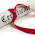### Problem

Evaluate the value of an arithmetic expression in Reverse Polish Notation. Valid operators are +, -, *, /. Each operand may be an integer or another expression.

For example:
```  ["2", "1", "+", "3", "*"] -> ((2 + 1) * 3) -> 9
["4", "13", "5", "/", "+"] -> (4 + (13 / 5)) -> 6
```

### Naive Approach

It can be solved using Stack
2. Push item into stack if it is number
3. Pop numbers from stack, if item is operator, do the operation for those numbers and push into the stack
```function evalRPN(tokens) {
var returnValue = 0;
var operators = "+-*/";

var stack = [];
for (var i=0; i<tokens.length; i++) {
if (operators.indexOf(tokens[i]) == -1) {
stack.push(tokens[i]);
} else {
var a = parseInt(stack.pop());
var b = parseInt(stack.pop());
switch (tokens[i]) {
case "+":
stack.push(a + b);
break;
case "-":
stack.push(b - a);
break;
case "*":
stack.push(a * b);
break;
case "/":
stack.push(b / a);
break;
}
}
}

returnValue = parseInt(stack.pop());

return returnValue;
}

console.log(evalRPN(['2','1','+','3','*']));
```

#### Complexity

This algorithm takes O(n) space and O(n) time

1.2.I like this post! The company https://topessaybrands.com/review/ewriters-pro-review/ has been around for more than a decade now. Within this time, they have brought more than 1,500 academic paper helpers on board. Among the papers that this platform handles include all types of essays (marketing, physics, nursing, chemistry, critical analysis, admission, etc.). They also handle other kinds of assignments like

## BlogrollSrinivas Dasari find me on facebook follow me on twitter